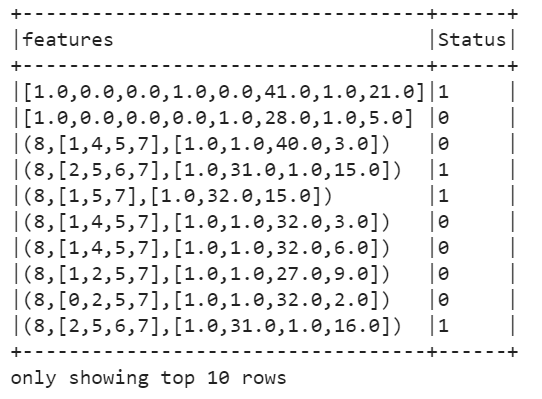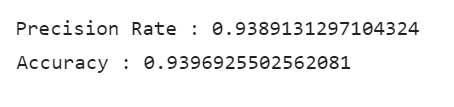top of page
Search

# Logistic Regression With PysparkIn statistics, logistic regression is a predictive analysis that is used to describe data. It is used to find the relationship between one dependent column and one or more independent columns. Dependent column means that we have to predict and an independent column means that we are used for the prediction.

Before building the logistic regression model we will discuss logistic regression, after that we will see how to apply Logistic Regression Classification on datasets using Pyspark.

Logistic regression

Logistic regression is the machine is one of the supervised machine learning algorithms which is used for classification to predict the discrete value outcomes. It uses the statistical approach to predict the outcomes of dependent variables based on the observation given in the dataset. There are three types of Logistic regression

1. Binomial Logistic Regression

2. Multinomial Logistic Regression

3. Ordinal Logistic Regression• It is simple and easy to implement machine learning algorithms yet provide great training efficiency in some cases. Due to this reason it does not require high computational power.

• This algorithm is proven to be very efficient when the dataset has features that are linearly separable.

• This algorithm allows models to be updated easily to reflect new data, ulike decision trees or support vector machines. The update can be done using stochastic gradient descent.

• Its outputs well-calibrated Probabilities along with classification results.

• It can't solve nonlinear problems with logistic regression since it has a linear decision surface.

• Logistic Regression is a statistical analysis model that attempts to predict precise probabilistic outcomes based on independent features. On high dimensional datasets, this may lead to the model being over-fit on the training set, which means overstating the accuracy of predictions on the training set and thus the model may not be able to predict accurate results on the test set. This usually happens in the case when the model is trained on little training data with lots of features.

Now here we are going build the Logistic regression model on the dataset using Pyspark

Why PySpark ?

• Spark is much faster.

• Spark is multi-threaded. It means two or more executions run concurrently. Whereas pandas are single threaded.

• Spark will only execute when you take Action.

So Lets Start..

Steps : -

1. Import some important libraries and create the SparkSession. SparkSession is the entry point of the program. Load the dataset search_engine.csv using pyspark.

Code snippet :

```import findspark
findspark.init()
#import SparkSession
import pyspark
from pyspark.sql import SparkSession
spark=SparkSession.builder.appName('Logistic_Regression').getOrCreate()

After loading the data when you run the code you will get the following result.

Output :PrintSchema : It displays the structure of data.Calculate Statistical data like Count, Average, Standard deviation, Minimum value, Maximum value for each column ( Exploratory Data analysis).

Code snippet :

```#statistical Data Analysis
df.describe().show()```Calculate total number of countries, platforms and status are present in datasets.

Code snippet :

```#count the country present in the datasets
df.groupBy('Country').count().show()

#count the search engine present in the datasets
df.groupBy('Platform').count().show()

# Count the status
df.groupBy('Status').count().show()```

Output :Lets see the visualization data.

Code Snippet :

```import matplotlib.pyplot as plt
import seaborn as sns
df11=df.toPandas()
sns.set_style('whitegrid')
sns.countplot(x='Country',hue='Platform',data=df11)```

Output :Categorical Data cannot deal with machine learning algorithms so we need to convert into numerical data. We use StringIndexer to encode a column of string categories to a column of indices and The ordering of the indices is done on the basis of popularity and the range. when you convert the column into numbers you will get the following result.

Code snippet :

```#import required libraries
from pyspark.ml.feature import StringIndexer

# Convert the platform columns to numerical
search_engine_indexer = StringIndexer(inputCol="Platform", outputCol="Platform_Num").fit(df)
df = search_engine_indexer.transform(df)

#Dsiplay the categorial column and numerical column
df.select(['Platform','Platform_Num']).show(10,False)
df.select(['Country','Country_Num']).show(10,False) ```

Output :Sometimes in a dataset, columns are found that do not have a specific number of preferences. The data in the column is usually shown by category or value of category and even when the data label in the column is encoded. So Now we are using OneHotEncoder to split the column which contains numerical data. when you split the column by using OneHotEncoder you will get the following result. We can see the platform column into the search_engine_vector column.

Code snippet :

```#import library onehotencoder
from pyspark.ml.feature import OneHotEncoder
#one hot encoding
search_engine_encoder = OneHotEncoder(inputCol="Platform_Num", outputCol="Search_Engine_Vector").fit(df)
df = search_engine_encoder.transform(df)```

Output :Now we are using VectorAssembler to concatenate the multiple columns into a vector column. It will combine all the features of multiple columns in one column. After applying the VectorAssembler we can see all the columns concatenated into feature columns.

Code snippet :

```#import vector assembler library
from pyspark.ml.feature import VectorAssembler

#concatenate all the columns in vector column.
df_assembler = VectorAssembler(inputCols=['Search_Engine_Vector','Country_Vector','Age', 'Repeat_Visitor','Web_pages_viewed'], outputCol="features")
df = df_assembler.transform(df)```

Output :Now Split your data into train and test data. Normally this is 70% and 30%. This method is used to measure the accuracy of the model.

apply the Logistic regression model. After applying the model you will get the following result. Status columns have original data, prediction column means it will predict the value calculated by this model and last column is the probability column.

Code snippet :

```#split the data
training_df,test_df=model_df.randomSplit([0.75,0.25])

#import the logistic regression
from pyspark.ml.classification import LogisticRegression

#Apply the logistic regression model
log_reg=LogisticRegression(labelCol='Status').fit(training_df)

#Training Results
train_results=log_reg.evaluate(training_df).predictions
train_results.filter(train_results['Status']==1).filter(train_results['prediction']==1).select(['Status','prediction','probability']).show(10,False)```

Output :Accuracy comes out to 0.9396. It obtains 93 % values that are correctly predicted by this model. That means our model is doing a great job identifying the Status.

Calculate the Precision Rate for our ML model. Precision Rate comes out to 0.9389. It means 93.89% Positive Predictions are correctly predicted.

Code snippet :

```#Calculate the matchine record out of the total records
accuracy=float((true_postives+true_negatives) /(results.count()))
print("Accuracy : " + str(accuracy))

recall = float(true_postives)/(true_postives + false_negatives)
print("Precision Rate : " + str(recall))```

Output :Thank you